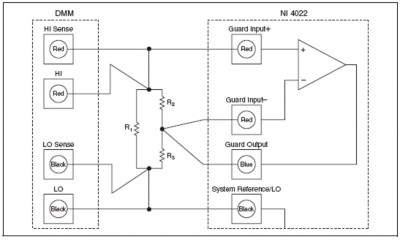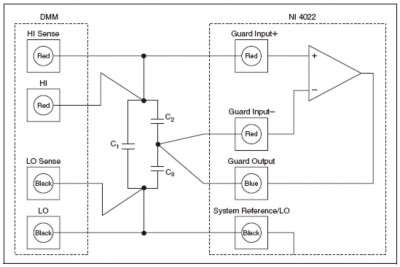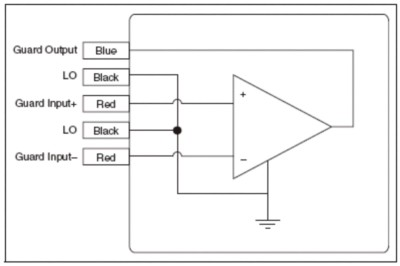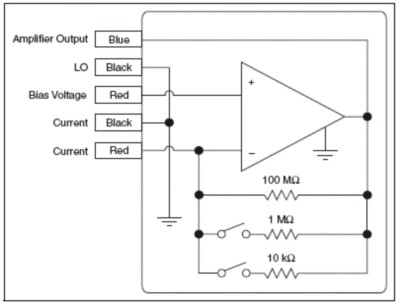# Improve Resistance Measurement Accuracy with 6-Wire Technique

## Overview

The concept of a basic resistance measurement is straight-forward: either source a known current across a resistor and measure the voltage drop or place a known voltage across a resistor and measure the current. These methods both work because of Ohm’s Law: V = I * R. However, testing methods become more difficult when the resistor under test is in parallel with another resistance or when automated test probes become contaminated from particles or solvents (and therefore provide a parallel resistance themselves). In either of these cases, some method of guarding the resistor under test is required. This practice is known as a 6-wire measurement.

For product information on NI digital multimeters and switches please see:
NI Digital Multimeter (DMM)
NI Switches

## Guarding Against Parallel Resistance or Contamination

When testing components on a printed circuit board (PCB), there will almost always be some level of resistance in parallel with the component in question. This challenge is faced by every in-circuit tester (ICT) or manufacturing defect analyzer (MDA) whose sole purpose is to isolate components on an assembled PCB to verify proper component placement and function. The problem can also exist on high-volume probe assemblies where contamination may build between contacts after hundreds or thousands of measurements. In these cases, the parallel resistance makes the Kelvin or 4-wire method of resistance measurements inadequate. To prevent the sourced current from traveling through one of the adjacent resistors, a unity-gain operational amplifier is inserted into the circuit (Figure 1), thereby guarding the resistor under test.Figure 1: Test connections for a guarded (6-wire) resistance measurement using a DMM and the NI PXI-4022 guard amplifier.

R1 is the resistor under test in the Figure 1. As the DMM sources the test current across it, some of that current would travel through R2 and R3 depending on their values. The configuration shown in Figure 1 uses the NI PXI-4022 guard and current amplifier module to apply a voltage between resistor R2 and resistor R3 that is equal to the voltage across R1. This applied voltage causes a voltage drop of zero across resistor R2. Thus, no current from the DMM flows through the path with resistors R2 and R3, effectively canceling the effects of these resistors from the measurement. A voltage exists across resistor R3 equal to the voltage across resistor R1, but that current is being supplied by the guard amplifier. In this sense, R1 is guarded. The same theory applies when the network consists of capacitors.

## System Consequences of Resistor Values

The resistance under test, R1, dictates the mode and range used on the DMM. This, along with the expected value of R1, determines the voltage that is driven across R1. To ensure proper operation of the guarding amplifier, this voltage must be used to calculate the minimum possible resistance that can be R3. For example, if R1 is 1 kΩ and is being measured on the 1kΩ range, a typical DMM would output a test current of 1 mA, generating a voltage of 1 V across R1. This same voltage appears across R3 when used with the guarding amplifier. Therefore, the amplifier must be capable of outputting enough current to equal the voltage divided by R3. If you need to guard out smaller values of R3, consider putting the DMM into a higher range. This will usually decrease the test voltage and the minimum value of R3 that will work in your system. The trade-off is that the measurement error of R1 increases as the values of R2 and R3 decrease.

Also, with the amplifier being non-ideal, its associated offset voltage will also contribute error when the value of R2 is small. The offset voltage of the guard amplifier is applied directly across R2 and the resulting current is subtracted from the calibrated test current from the DMM (which is meant to flow through R1), causing an error. For example, in the earlier case measuring a 1 kΩ resistor on the 1 kΩ range with the 1 mA test current, if R2 is 100 Ω and the typical offset voltage is 200 μV, the error in the test current is 200 μV/100 Ω, or 0.002 mA, which steals 0.2% of the 1 mA test current. This results in a measurement error on R1 of 0.2%. These additional errors are straightforward to calculate and must be added to the total system error.

 For R 1 = 1kΩ, R 2 = 8.2kΩ, R 3 = 4.3kΩ 4-Wire Measurement 6-Wire Measurement with NI PXI-4022 Test Current 1 mA 1 mA Guard Offset Voltage N/A 200 µV Current Across R 1 926 µA 999.976 µA Current Across R 2 74 µA .024 µA Current Across R 3 74 µA 23.255 µA Measured Value of R1 926 Ω 999.9 Ω Measurement Error -7.4% -.01%

## Embedded Capacitance Measurements

The NI PXIe-4082 FlexDMM is an LCR meter with a capacitance measurement range from 300pF to 10,000uF . The same 6-wire measurement technique can be used with capacitance measurements. By once again enabling a common potential across a capacitor, the guard card effectively cancels out the affects of parallel capacitance. This gives the PXI-4072 the ability to take accurate measurements of embedded capacitors.Figure 2: Test connections for a guarded (6-wire) capacitance measurement using the NI PXIe-4082 FlexDMM with LCR Meter and the NI PXI-4022 guard amplifier.

## Additional Information on the NI PXI-4022 Guard and Current Amplifier Module

The basic structure of the NI PXI-4022 is a unity gain amplifier that can also function as a preamplifier for making low-level current measurements. Figure 3 is a block diagram for the guard mode showing the circuit relative to the input and output connectors. Figure 4 shows the block diagram for the other mode of the PXI-4022 - current amplifier mode.Figure 3: Guard mode for the NI PXI-4022.Figure 4: Low-current preamplifier mode for the NI PXI-4022.

More information can be found on the low-current preamplifier mode in the specifications document for the NI PXI-4022.